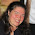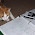## 1.04.2013

### #myfavfriday Slope Formula

I'll go ahead and warn you that this won't be the best explanation.

I'm posting about a different way (for me) of teaching the slope formula. I transition from functions to linear functions via tables. As we learn to use a function rule to create a table, we then plot the points in the table and graph.

After we are experienced with that, I teach about slope (I should blog about that too I suppose) in context and we start counting rate of change and slope.

Once we're familiar with that concept, I take students back to tables and show them how to find the slope from a table. For the first time, I translated that to ordered pairs. When I give the student's ordered pairs, they write them in a table (t-chart) and subtract to find the slope.

Here's an example:

I like this way better because there isn't as much confusion in finding x1 and y1. It doesn't matter which ordered pair you write first anyway.

Sometimes the students do write the pairs vertically instead of horizontally but overall I am much more satisfied with this method rather than the formula.

As much as I can, I am trying to get away from memorizing formulas and trying to build things conceptually. Now you may say that students have to memorize this method as well but I would respond to that that the tables are much more of a natural progression than a random formula.

Na na boo boo.

1.I like this. My students really struggled with the slope formula. Definitely going to try this next year!

1.2.Hi I'm a new follower. Thanks for sharing all your awesome ideas.

I changed how I taught finding slope this year, too. I started with rate of change based on money vs. time in a word problem. Then I made a chart for a similar money vs. time scenario. We talked about finding rate of change as the ratio of the difference in the dependent to the difference of the independent and how that works in any xy-chart. When we plotted these points I related it to the rise over run slope activity we had done the week before and they could now "measure it" on a graph. I loved how I never once had to mention y2-y1. They are so much better at finding slope than my students have been in the past because of this new approach!

3.Thank you for sharing the sequence in which you teach slope! We also taught how to find the rate of change, aka slope, through tables before we introduced the formula. As we progressed through our unit on linear equations, students used both methods - many of them choosing the tables method over the formula!

4.I like this approach. Thanks for sharing. It is much more straightforward and gets around the x1 - x2 stuff which is confusing.

5.I've used this method for years - actually it came out of a discussion during my NBCT over 10 years ago. a former student called it "the street method" lol when I told them it wasn't the traditional textbook forumula. I prefer this "strategy" bc it ties to using 1st differences for (linear) function families. Also, I keep a vertical line in my room to help students decided if they're moving "+" or "-". Hope it works as well with your students as it has with mine!

6.I love this method too! I teach an alternative math class, which often explains the "why" behind certain concepts (like slope). Now, in my traditional Algebra class, I'm not even teaching the formula. This just clicks more!

7.In the past, I taught a "Stack them and Subtract them" method similar to this. I just had the kids stack the ordered pairs and then subtract the two numbers, remembering to put the final answer y divided by x. I really liked that way and this way. I never cared for the formula.

1.And I just used this to teach the distance formula without the formula. It worked soooo much better even though they still screw up with negatives.

8.Love it! I had my students work in groups to develop a formula (which for the most part ended up matching the traditional formula), but I still have a few students who continue to either mix up rise/run or switch the numbers around...this will be a good way for them to try, or to justify their answers. Thanks!

9.This is a great way to teach slope! I tutor math at a university (taking my teaching certification test for high school later this month) and this would have been sooooo much easier to get across to the students. (A lot of the students that come for tutoring have not had to use their high school math kills for a while so they are a tiny bit rusty.)

BTW I found your blog via Pinterest and I am loving your ideas and suggestions.

10.Yay! I must have made it to the big leagues if you found me on Pinterest...lol. Welcome =)